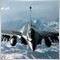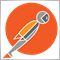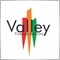# get last closed order lot size366

Hello  every one

the Below Function is to get the Last order lot size and multiply it by 2 it is is lose trade but it is not working properly , can you help

```double fLotSize()

{

double fnewlot=Lot_size;

int i =OrdersHistoryTotal();

for (i;i>=0;i--)

{

if (OrderSelect(i,SELECT_BY_POS,MODE_HISTORY)==false){GetLastError();}

{

if (OrderSymbol() == Symbol() && OrderMagicNumber() == magic_no)

{

{

if (OrderProfit()<0){(fnewlot=(OrderLots()*2));}

}

else if ( OrderProfit()>0){(fnewlot=Lot_size);}

}

else (fnewlot=Lot_size);

}

}

return(fnewlot);

}

```2392

Ahmed Tawfik:

Hello  every one

the Below Function is to get the Last order lot size and multiply it by 2 it is is lose trade but it is not working properly , can you help

double fLotSize()

{

double fnewlot=Lot_size;

int i =OrdersHistoryTotal();

for (i;i>=0;i--)

{

if (OrderSelect(i,SELECT_BY_POS,MODE_HISTORY)==false){GetLastError();}

{

if (OrderSymbol() == Symbol() && OrderMagicNumber() == magic_no)

{

{

if (OrderProfit()<0){(fnewlot=(OrderLots()*2));}

}

else if ( OrderProfit()>0){(fnewlot=Lot_size);}

}

else (fnewlot=Lot_size);

}

}

return(fnewlot);

}

First off, what does the last order mean? The last opened order? The last closed order? Next you need to sort them programmatically in order to work with them. So for example if you said, "my criteria for 'last order' really means the most recently opened order", then you would need to sort the history pool by OrderOpenTime. Below is an example using the MQL4OrderPool class. BTW, next time you post code, please use the SRC button.

```int LotSize(int magic,double start_lot_size)
{
MQL4OrderPool pool;
pool.Init(Symbol(),magic,MODE_HISTORY,ORDERS_FILLED,SORT_OPEN_TIME_DESCENDING);
int losing = 0;
if(pool.Total()==0)
return start_lot_size;
return NormalizeDouble(pool.OrderLots() * 2, 2);
}```
Files:366

first of all thanks ,

2nd this is my first post ever here , i will use the SRC next Time

It took me long time to find My post again :)

Yes , what I meant my last order , is the most recent closed order

as for the code can you explain how it works , thanks48

hey guys, please give me a light,

i'm with a trouble with getting the ordersize that was opened,

my ea works with a "switch"

if RSI (10) > 70     - i want to use my baselotsize / 2. [ buy case ]

if RSI (10) < 70     - baselotsize;  [ buy case ]

if RSI (10) < 30      -i want to use my baselotsize / 2. [ sell case ]

if RSI (10) > 30      - baselotsize;  [ buy case ]

i use martingale with loose blocks on code. i need to "read" the lotsize after the opening of the first order and store this, how do i do that?